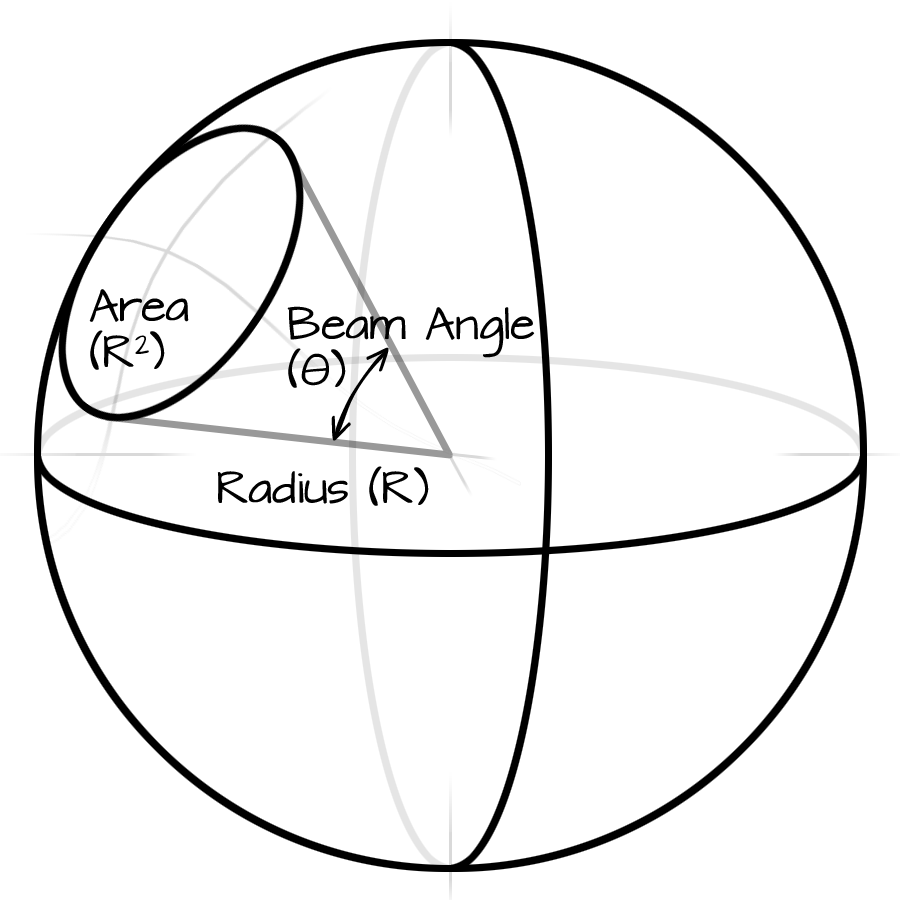# mcd to Lumens Converter

This calculator is useful for calculating the luminous flux for LEDS where the angle is known.

1 candela of luminous intensity equals 1 lumen of luminous flux per steradian of solid angle, where a steradian respresents a cone whose surface area at the end equals the length of the cone squared.where:

• Φv is the luminous flux, in measured in Lumens (lm),
• Iv is the luminous intensity, measured in Candela (cd), and
• Ω is the solid angle of the beam is measured in Steradian (sr).

Conversion from beam angle to solid angle (sterians) is done with this formula:where:

• θ is the the beam angle measured in degrees.Enter any two known values and press "Calculate" to solve for the other.

millicandelas (mcd)
Degrees
Lumens (lm)
Click "Calculate" to update the field with orange border.